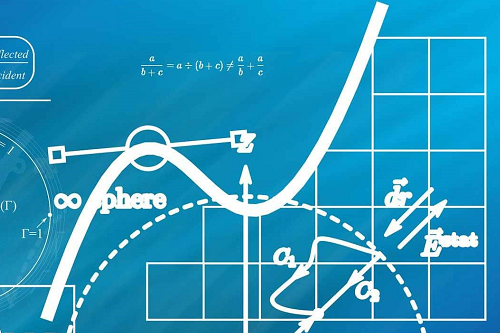#### A Level English Sprint Finish#### A Level Maths Sprint Finish

Covering all exam boards and Pure Mathematics, Mechanics, and Statistics modules.

A level mathematics is usually divided into three subject areas. The core modules are termed “pure mathematics”. The other modules cover mathematics applied to mechanics and mathematics applied to statistics. Most students will be able to choose which applied module to take, although some schools choose which to offer and for a few examining-boards, students study both.

The mechanics module will mostly interest students aiming at the physical sciences or engineering. The statistics module will be a good grounding for social scientists and business analysts.

Core modules will include most of the following; algebra, quadratic equations, functions, graphs, geometry, trigonometry, vectors, differentiation, integration, logarithms, roots, exponents, indices, complex numbers, surds and polynomials.

In the mechanics module you will study; forces and equilibrium, moments, vectors, scalars, coefficient of friction, Newton’s laws of motion, acceleration, energy, power, resistance, compression, elasticity and Hooke’s Law.

The statistics module will introduce you to; probability, stem and leaf diagrams, histograms, box-and-whisker plots, means and medians, variance, standard deviation, permutations, normal and binomial distributions, Poisson distribution, sampling, Central Limit Theorem, one and two tail tests, hypothesis construction, significance-levels, sum and product laws, scatter diagrams and linear regression.#### A Level Physics Sprint Finish